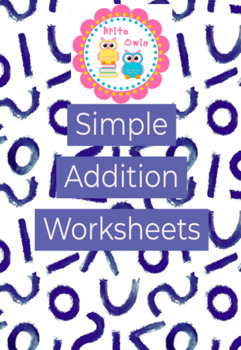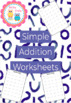# Simple Addition Worksheets - Basic Math FactsSubject
Resource Type
File Type

PDF

(1 MB|33 pages)
Product Rating
Standards
• Product Description
• StandardsNEW

This 30-page file contains "mad minute" style addition facts for numbers 0-10

I gave my students every week one page in a protector sheet with dry erase & a timer to practice over and over to build fluency.

You can also use it as a daily sheet, time filling in the class or send it as HW.

Enjoy!

Determine the unknown whole number in an addition or subtraction equation relating three whole numbers. For example, determine the unknown number that makes the equation true in each of the equations 8 + ? = 11, 5 = ▯ - 3, 6 + 6 = ▯.
Understand the meaning of the equal sign, and determine if equations involving addition and subtraction are true or false. For example, which of the following equations are true and which are false? 6 = 6, 7 = 8 - 1, 5 + 2 = 2 + 5, 4 + 1 = 5 + 2.
Total Pages
33 pages
N/A
Teaching Duration
30 minutes
Report this Resource to TpT
Reported resources will be reviewed by our team. Report this resource to let us know if this resource violates TpT’s content guidelines.